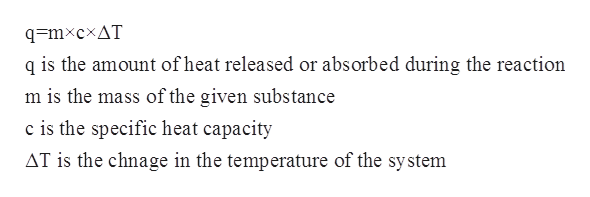# A 15-gram sample of lead (c = 0.128 J/g degrees celsius) is heated from 25 degrees celsius to 215 degrees celsius. How much heat is absorbed?

Question
56 views

A 15-gram sample of lead (c = 0.128 J/g degrees celsius) is heated from 25 degrees celsius to 215 degrees celsius. How much heat is absorbed?

check_circle

Step 1

The specific heat capacity for a given substance refers to the amount of heat that is needed to raise the temperature of a given substance per unit of its mass. The relationship between specific heat capacity, mass, change in temperature and the heat of the solution is given by the following equation:help_outlineImage Transcriptioncloseq=mxcxAT q is the amount of heat released or absorbed during the reaction m is the mass of the given substance c is the specific heat capacity AT is the chnage in the temperature of the system fullscreen
Step 2

In this problem, it has been given that the mass of the sample of lead is 15 g.

The change in temperature is given to be from 25oC to 215oC which can be calculated as follows:

Step 3

The specific heat capacity of the sample of lead is given to be 0.128 J/g oC

S...

### Want to see the full answer?

See Solution

#### Want to see this answer and more?

Solutions are written by subject experts who are available 24/7. Questions are typically answered within 1 hour.*

See Solution
*Response times may vary by subject and question.
Tagged in

### Physical Chemistry